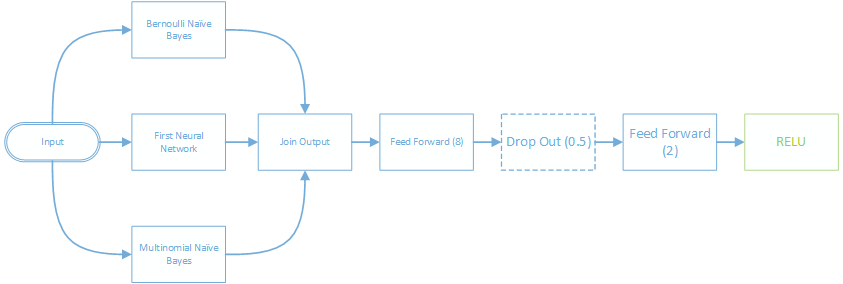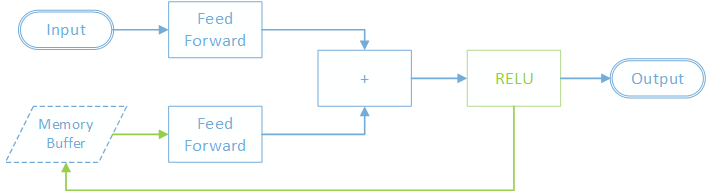# Project overview and quick guide to getting started.

Bright Wire is an open source machine learning library for .NET with GPU support.

In other words:

• It's free and open source - you're free to use it in any commercial or personal project.
• It includes the most commonly used machine learning algorithms such as Linear Regression, Logistic Regression, Naive Bayes and Neural Networks (feed forward, convolutional and recurrent).
• It runs on .NET Core which means it can run on Windows, OS X, Linux etc.
• Most included machine learning algorithms can be trained on the GPU for improved learning performance.

Bright Wire is an end to end machine learning solution, covering both model training and efficient model execution in production. Once your machine learning models have been trained you can execute them using highly optimized linear algebra libraries such as the Intel MKL library - or the GPU - all from within the same library.

### Graph Oriented

Bright Wire is oriented around nodes and wires (edges) that form a directed graph. Input data is fed into the graph and wires (edges) carry the signals around the graph.Nodes can calculate an error signal (such as via back propagation) that flows back across the graph in reverse. Prior nodes can use the error signal to update their parameters before sending the error signal further backwards along the graph. This is the learning part of Bright Wire.

### Easily Extensible

It's simple enough to create your own new nodes that you can feed into the graph. It's also easy to combine existing nodes into novel network architectures. Bright Wire makes it easy to experiment with machine learning with the performance and type safety of managed languages such as c#.

For example, a recurrent neural network node is implemented with a sub graph of nodes as follows:Treating a machine learning algorithm as a composition of nodes makes machine learning almost analogous to snapping together LEGO bricks.

### GPU Training

Bright Wire supports GPU based machine learning by implementing every linear algebra operation twice - once for the CPU and once for the GPU. This means that any machine learning algorithm that uses linear algebra can run on either the CPU or GPU.

## Installing from NuGet

### .NET Core

The .NET core version is installed with:

### .NET 4

The base library includes all machine learning algorithms and CPU based linear algebra:

If you have a Keplar or better NVIDIA GPU and have installed Cuda Toolkit then you can add GPU support with:

## Data Tables

The data table is Bright Wire's workhorse data type. Data tables are the single way to describe data for machine learning in BrightWire.

• Once built, Data Tables are immutable.
• Contains rows of data with each row having the exact same format.
• Each column has a distinct data type, such as string, number, date or boolean.
• Optionally includes a labelled classification target column.
• Either stored in memory or the file system.
• Can be analysed to find column means, variances etc.
• Data tables can contain vector, matrices, 3D tensors, index lists and weighted index lists.
• Different machine learning algorithms expect data tables with different column types.

### Data Table Column Types

The type of each column can be as simple as a single number, and as complex as a 3D tensor (a cube of numbers). If a column is a string it will automatically be treated as a categorical column and one hot encoded.

#### Index Lists and Weighted Index Lists

Some machine learning algorithms expect input in the form of a list of numbers with an associated classification label (for example the multinomial naive bayes classifier). This is expressed with a two column data table - the first column is of type Index List and the second (the classification target) is of type string. Each row in the table is a training example.

#### Matrix and Vector Columns

Neural networks expect some variant of vectors. A simple feedforward neural network expects a table with two columns, each of type Vector. The neural network learns to map each input vector to the output (classification target) vector.

A recurrent neural network expects an input column of type Matrix. Each row in the matrix is an item in the input sequence. It expects the second column (the classification label) to be of type Vector. So each matrix/vector pair is a training item.

Finally a convolutional neural network expects an input column type of 3DTensor and output column of type Vector.

## Learning More